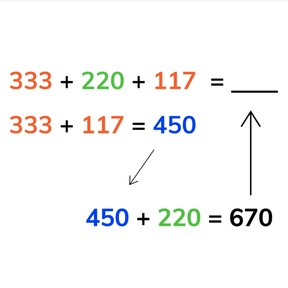8,000 schools use Gynzy92,000 teachers use Gynzy1,600,000 students use Gynzy

## General

Students learn to add to 1,000 using grouping of three or more addends. They learn to first find a pair or pairs of addends that total a round number, and then to add the final addend(s).

2.NBT.B.7

## Relevance

It is important that students are able to use grouping to add to 1,000 so that they can quickly and easily add three or more addends.

## Introduction

Students are shown a number line to 1,000. Show that Brandon is at 530. Ask how you can calculate how many steps Brandon must take to get home. Explain that it is useful to first go to the next hundreds, then tens, and finally ones.

## Development

Explain that tens are a group of ten have a zero in the ones place, and that hundreds are groups of 100 and have zeros in the tens and ones places, and that both of them are round numbers. Say that when you add with three or more addends, you look for two addends to total a round number to make you addition easier.
With addition problems with four addends, show students that you first make two pairs of addends: 376+124 and 235+215. In the last step you show that you add the totals of the prior step together to find the total. Practice this again with students, going through all of the steps.
Next show students how they can solve an addition problem with four addends in a table. Explain that you first make two pairs of addends that total a round number- make sure to be clear that these numbers do not have to be across from each other, or above/below each other- they must look closely at all the addends. Students are asked to add some addition problems in table form.

Check that students are able to add to 1,000 using grouping by asking the following questions:
- How do you add by grouping?
- What do you pay attention to when grouping addends?
- What do you pay attention to while adding?
- Which steps do you take when adding three or more addends with grouping?
- Do you always group the first two addends?

## Closing

Check that students have understood the intstructions by asking them to solve a few addition problems with three or more addends to 1,000. To close the lesson, ask the students to form pairs. Explain to the pairs that you have a bridge that can take 1,000 pounds. They must determine how many trucks and cars are able to be on the bridge at once. If the total is more than 1,000 the bridge will break. Challenge the pairs to see who can determine the most trucks and cars that are able to be on the bridge at once. Ask students to write the numbers and their totals on a sheet of paper to keep track of their thinking.

## Teaching tips

Students who have difficulty determining the order in which they should add with three or more addends should practice adding to tens and hundreds first. They can use MAB-blocks or manipulatives to set out the numbers. They can then select an appropriate number that would create a round number.

## Instruction materials

Optional: MAB blocks

### The online teaching platform for interactive whiteboards and displays in schools

• Save time building lessons

• Manage the classroom more efficiently

• Increase student engagement